CHEMISTRY

 Time: 1 Hour Max.Marks : 60

Instructions:
(1) Answer must be written either in English or the medium of instruction of the candidate in high school.
(2) There will be no negative marking
(3) Use of calculators or graph papers is not permitted
(4) There are TEN questions. Answer all the questions.

 1. Chromatography is used by the ‘Horse Racing Forensic Laboratory’ to test for the presence of illegal drugs in racehorses. A concentrated sample of urine is spotted onto chromatography paper on the start line. Alongside this, known drugs are spotted. The chromatogram is run using methanol as the solvent. When finished , the paper is read by placing under ultra-violet light. A chromatogram of urine from four racehorses is shown.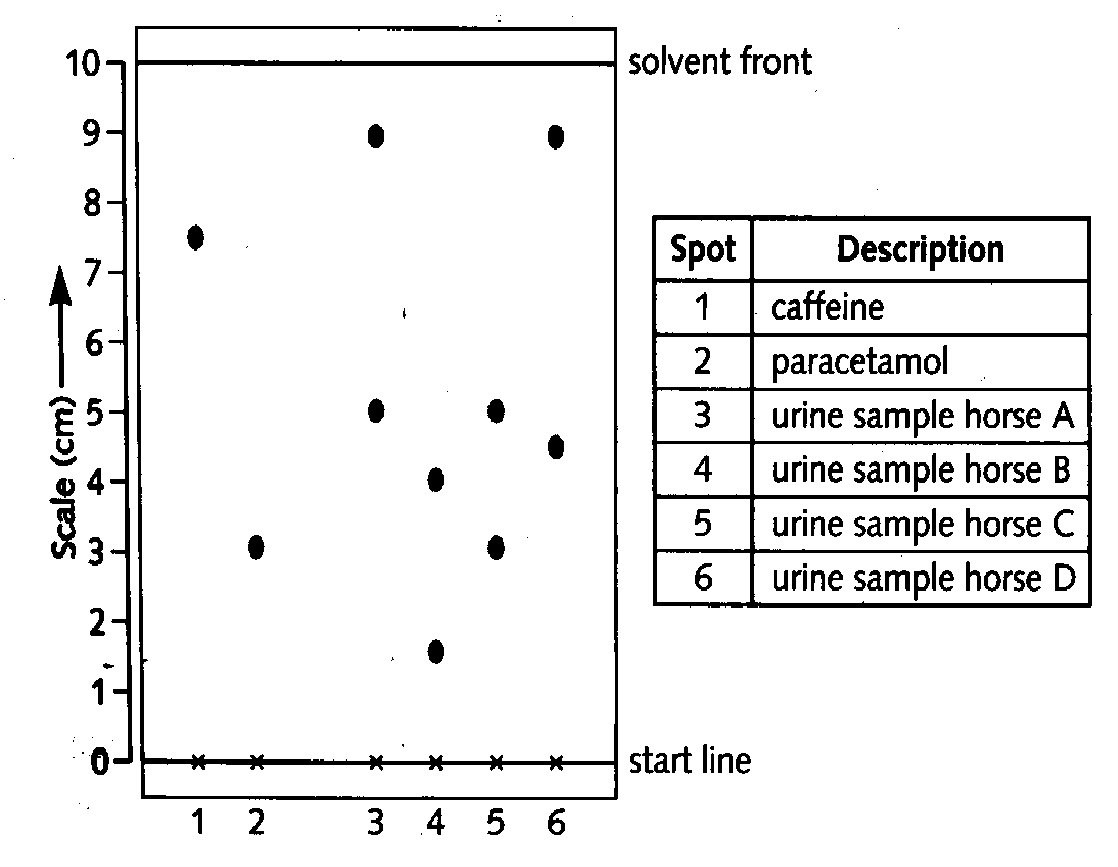a) The results for known drugs are given as ‘Rf values’.;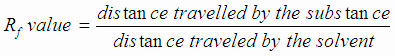calculate the Rf value for caffine ; 1M

b) Explain the meaning of the word ‘solvent’ ; 1M

c) State two factors which determine the distance a substance travels up the paper. 1M

d) From the results the sample from one horse contains an illegal substance.

i) State the horse, and the drug present.; 1M

ii) Give a reason for the use of this drug. ; 1M

2. Some solids dissolve easily, others do not

a) i) What is the name given to liquids which dissolve solids? ; 1M

ii) What is the name of the clear liquid formed when a solid dissolves in a liquid? 1M

b) Temperature change affects the solubility of substances in water.

i) How does the solubility of most solids change as the temperature of the water increases? 1M

ii) How does the solubility of a gas change as the temperature of the water increases? 1M

c) Describe how dry solid can be obtained from the liquid in which it is dissolved. 1M

 d) The graph shows how the solubility fo ammonia sulphate in water changes with temperature. 100g of ammonium sulphate was heated in 100g of water until it all dissolved. Use the graph to answer the following. i) What is the lowest temperature at which the water will dissolve all the ammonium sulphate? 1M ii) What mass of solid will crystallizes from the liquid when it is cooled to room temperature? 1M iii) This solid is filtered off at 20oC. what will you see if the remaining liquid is then cooled to15oC? 1M iv) What word is used to describe a liquid which cannot dissolve any more solid at a given temperature? 1M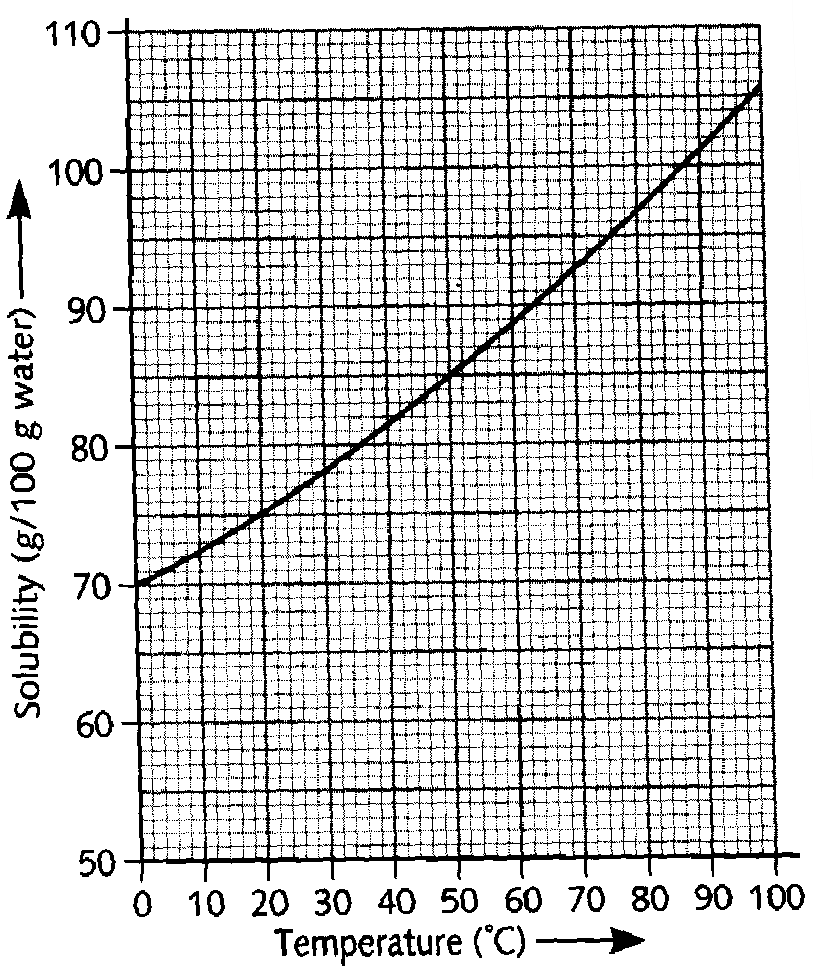3. Write balanced chemical equations for the reactions between: ; 5M

i) Sodium thiosulphate and hydrochloric acid

ii) Magnesium and steam

iii) calcium and oxygen

iv) Bromine and potassium iodide solution

v) Zinc and copper sulphate solution.

 4. Magnesium is obtained by the electrolysis of magnesium chloride in a cell similar to the one shown below. a) i) What is cathode made from ? 1M ii) What is the anode made from ? 1M  iii) Why is the chlorine gas kept separate from the magnesium metal? ; 1M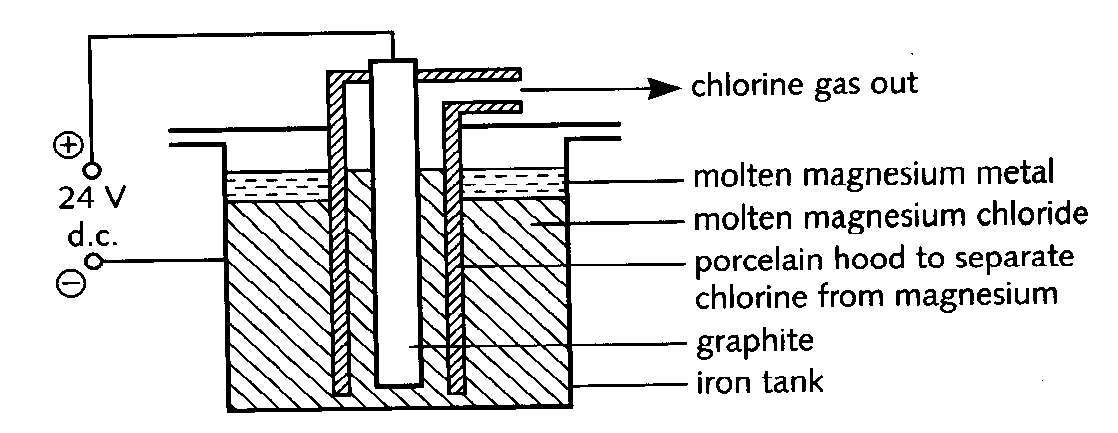b) The magnesium chloride is made by the following reaction ; 1M

MgO + C+ Cl2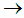MgCl2 + CO

Rewrite the equation in words

c) Name two other metals that are obtained by electrolysis ; 1M

5. Reactions that give off carbon dioxide are used to raise dough when baking bread. On cooking, the bubbles of carbon dioxide become trapped in the dough and give the bread a pleasing texture.
The ingredients are made into a dough which is left in a warm place for about 30 minutes. The volume of the dough increases as carbon dioxide is produced from the sugar by the yeast.
a) There are two ionic compounds in the recipe. Name one of them. ; 1M The dough is then placed in a hot oven and the baking powder produces more carbon dixode as the naan bread cooks

 Recipe card NAAN INDIAN BREAD Ingredients 75 cm3 of milk 1 teaspoon of sugar 1 teaspoon of yeast 200g of plain flour ½ teaspoon of baking powder (sodium hydrogencarbonate and tartaric acid) 1 tablespoon of vegetable oil 75 cm3 of plain yogurt 1 egg ¼ teaspoon of salt

Sodium hydrogen carbonate decomposes when heated.

2NaHCO3Na2CO3 + CO2 + H2O

Sodium carbonate has an unpleasant taste. It reacts with the tartaric acid to form sodium tartarate which has a pleasant flavour.

b) What type of compound is sodium tartarate? ; 1M

c) Suggest two reasons why baking powder is a mixture of tartaric acid and sodium hydrogen carbonate, and not just sodium hydrogen carbonate. ; 1M

6. A packet of washing soda crystals has been left open to the atmosphere for some time. The crystals have formed a white powder which may be represented by the formula Na2CO3. xH2O. The value of ‘x’ may be found by dissolving a known quantity of the powder in water and titrating the solution with acid of known concentration.

In one experiment a student weighed out 1.59g of the powder and dissolved it in water. The volume of the solution was made up to exactly 250cm3. 25cm3 of this solution was titrated with hydrochloric acid of concentration 0.10 mol/dm3 (mol per litre). 25.6 cm3 of the acid was required for complete reaction. The equation for this reaction is:

Na2CO3 + 2HCl2NaCl + H2O + CO2

a) Calculate the number of moles of hydrochloric acid used in the titration. ; 1M

b) Calculate the number of moles of sodium carbonate in the 25cm3 sample of solution which reacted with the hydrochloric acid. ; 2M

c) How many moles of sodium carbonate were in the 250cm3 of solution? ; 1M

d) Calculate the mass of sodium carbonate in the 250cm3 of solution. ; 1M

(Relative atomic masses C= 12, O = 16, Na = 23)

e) Using your answer to part (d), what mass of water was in the original powder? ; 1M

f) How many moles of water were present in the original powder ?

(Relative atomic masses H = 1, O = 16) ; 1M

g) Using your answers to parts (c) and (f), calculate the value of x. ; 2M

7. a) The Haber process is a reversible reaction. What does ‘reversible reaction’ mean? 1M

b) The graphs show the amount of ammonia produced at different temperatures and pressures.

i) What conditions favor the production of ammonia? 1M

ii) In industry the reaction is carried out at 450oC and 200 atmospheres pressure. Use the graph to explain why these conditions are used. 2M

c) i) Some energy has to be put into the chemicals to start the reaction. Explain how this energy helps the reaction to start. 2M

 ii) Here is an energy level diagram for this reaction. Is this reaction exothermic or endothermic? Explain your answer in detail 1M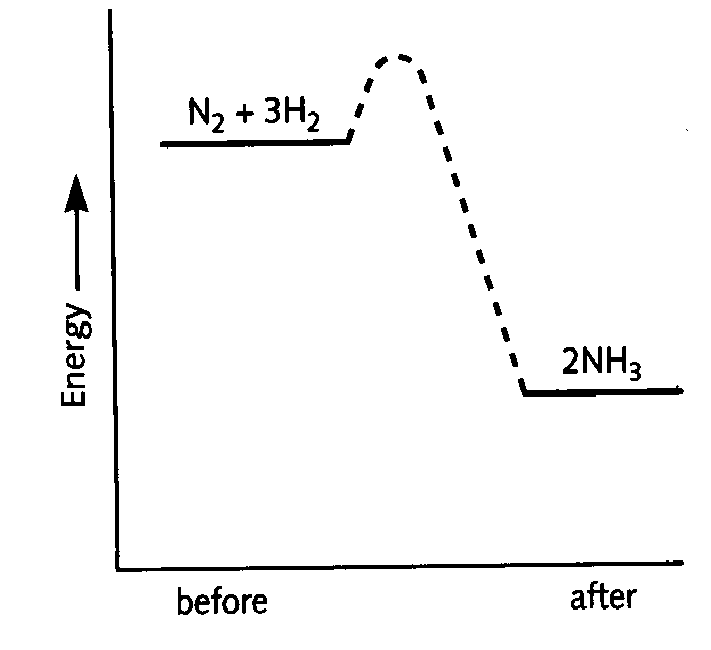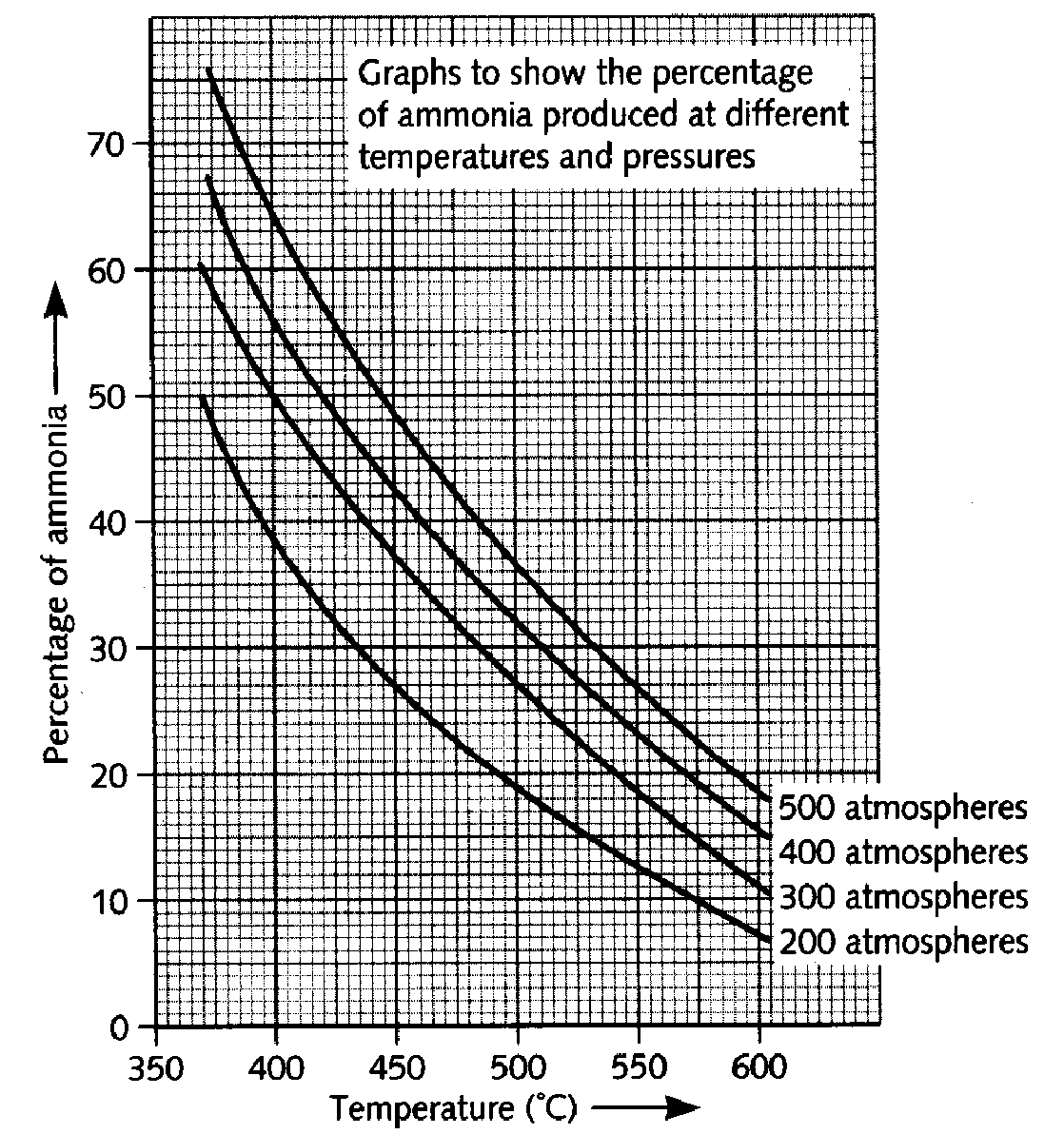8. This question is about alcohols.
a) The alcohols are members of a homologous series.
i) Write down the general formula for this homologous series. ; 1M

ii) Write down the names and draw the structures of the two monohydric alcohols (alcohols that have only one –OH group) containing three carbon atoms per molecule ; 1M

b) On analysis, a 10.00g sample of an alcohol was found to contain 3.75g of carbon, 1.25g of hydrogen, and 5.00g of oxygen. Use this data to find the empirical formula of this alcohol. Suggest its molecular formula. ; 2M

c) The dissociation energy of ethanol is shown below

C2H5OH(g)2C(g) + 6H(g) + O(g) D H = + 3234 kJ/mol of ethanol

Using bond energy values (given below) calculate the bond energy of C-O per mole. 1M

[Bond energies : H - H : 436 kJ/mol , C- H : 435kJ/mol, O- H : 464 kJ/mol, C- C : 347 kJ/mol]

9. Red Lead, Pb3O4 is used in paints. It protects metal surfaces against corrosion. Red lead is made by heating lead (II) oxide, PbO, in air.

a) copy out and balance the following equation ; 1M
PbO + O2Pb3O4

b) Red lead paint may be used to protect iron from rusting. Name two other ways of stopping iron or steel from rusting. ; 1M

c) On strong heating, red lead decomposes to form oxygen and lead (II) oxide.

i) How could you show that oxygen is given off in the decomposition? ; 1M

ii) Suggest how you could show when the decomposition was finished ; 1M

d) Red lead reacts with concentrated hydrochloric acid to form lead (II) chloride , chlorine and water. Pb3O4 + 8HCl3PbCl2 + Cl2 + 4H2O

i) Describe how you could show that chlorine was given off during the reaction. ; 1M

ii) Suggest how, using an electrical method, you could obtain lead from lead (II) chloride. 1M

 10. The rate of reaction between a metal and bromine can be studied using the apparatus shown below. A piece of metal, for example iron, was weighed and placed in the solution as shown above. The metal was removed at regular intervals and each time it was washed, dried and weighed. It was then replaced in the solution and the reaction continued. From the results (mass of metal and time), the rate of the reaction can be calculated. a) i) How would the rate change if more of the piece of metal was in the solution? Explain your answer ; 1M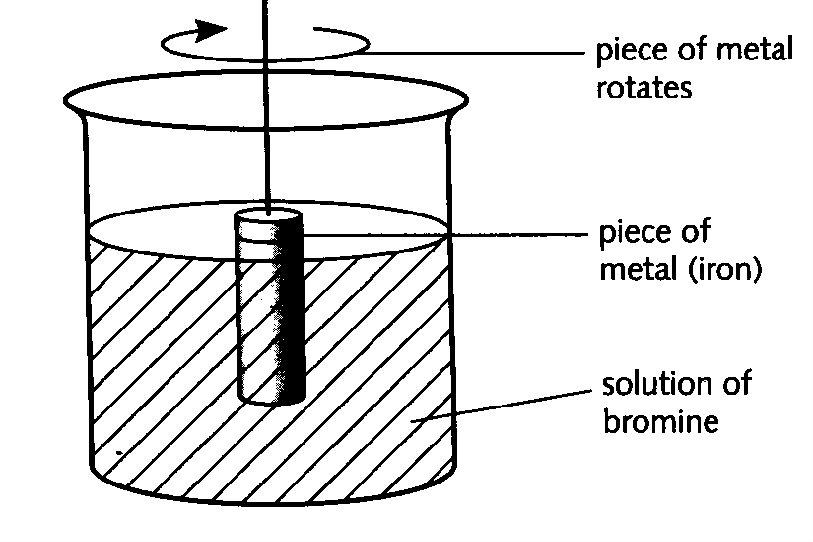Concentration of bromine solutions Rate 0.05 mol/dm3 10mg/minute 0.1 mol/dm3 20 mg/minute

Explain the change in rate. ; 1M

b) In a reaction between a solid and a solution, the rate may depend on the speed of stirring. Describe how you could find out if the rate of the reaction between solid iron and bromine solution depends on the speed of stirring. ; 1M

c) Iron has two oxidation states so it can form two ions: Fe2+ and Fe3+

How could you test a solution to find out which ion is present? Outline the test, and give the results for both ions. ; 1M

d) In the reaction between iron and bromine: iron atoms change into iron ions; Bromine molecules change into bromide ions.

i) Which of these changes is a reduction? Explain your choice. ; 1M

ii) If iodine was used instead of bromine, predict how the rate of the reaction would change: increase, decrease or stay the same. Give a reason for your answer. ; 1M